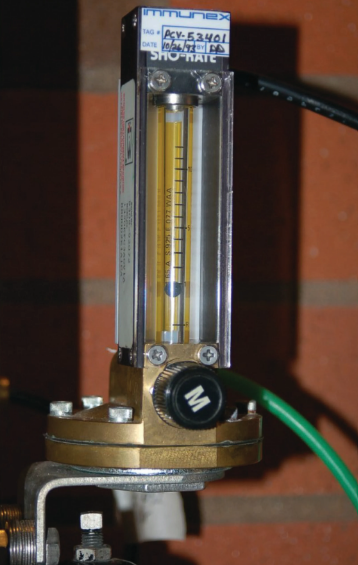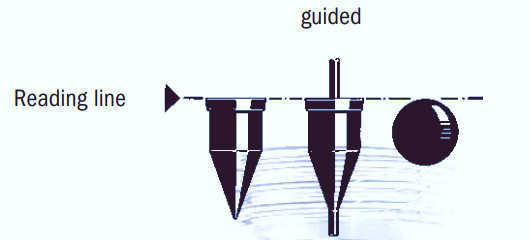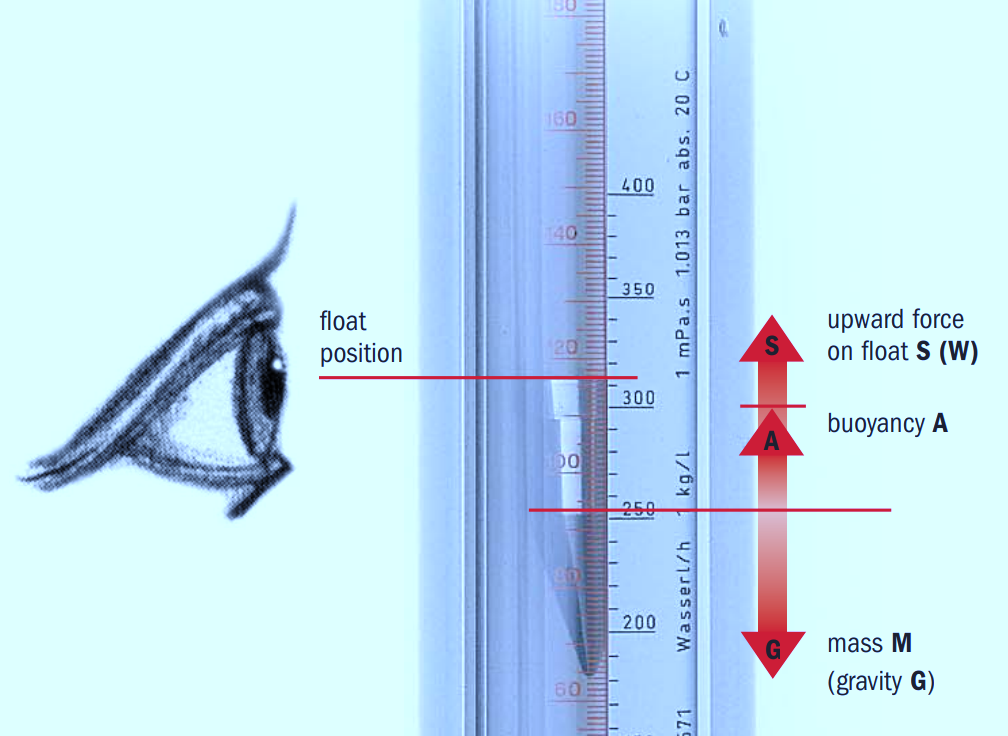Flow Measurement

# Variable Area Flow MeterIn this article, we will study the working of Variable Area Flowmeters.

## Variable Area Flow Meters Principle & Operation:

• This flow meter operates on the variable area principle.
• This Variable area flow meter works at a constant Delta Pressure (Δp).
• Cone & Float type is the most common design of variable area flow meter which is also known as a Rota meter.
• Basically, the design of a variable area flow meter is a tapered glass tube with a self-centering float pushed upwards by the fluid flow and pulled downwards by the action of gravitation.
• At maximum flow rates, the float rises upwards to enlarge the area between the float and tube to maintain a constant Delta Pressure (Δp).
• The float shifts up or settles down in the tube in direct proportion to the flow rate of fluid, and the annular area between the float and the tube wall.
• The flowing fluid varies the position of a float, piston, or vane to make the area large for the passage of the fluid.
• The position of the float, piston, or vane gives a direct visual indication of the flow rate.
• The flow rate can be calculated by the amount of rise of the float inside the tube.
• In the case of liquid, the float is raised by a combination of velocity and buoyancy of fluid.
• Whereas in the case of gas, this buoyancy is negligible, and the float responds only to the velocity head.
• Variable area flow meters can be used as indicators, transmitters, recorders, local controllers, totalizers, and many other combinations with or without alarms.

## How to determine the flow rate of fluid in Variable Area Flow Meters?

The flow rate can be calculated by using the following relation

Q = k*A*√ (g*h)

Where:

Q = flow rate in GPM

k = a constant

A = Area

g = force of gravity

h = head across the float

## How Does the Variable Area Flow Meter Work?

• The fluid may be liquid, steam, or any gas that flows through the inverted tapered glass tube from the bottom to the top carrying the float upwards as indicated by the arrow.
• The diameter of the glass tube goes on increasing in the upward direction due to the tapered structure.
• The float rises to a certain point where the upward force on the float gets exerted by DP across the annular gap between the float and the tube equals the float weight.
• Three forces act on the float are
• Constant Gravitational Force, W.
• Buoyancy Force, A,
• Flow Resistance Force S
• According to Archimedes principle, buoyancy force A remains unchanged if the fluid density is constant
• Flow Resistance Force S is the upward force of the fluid flowing past the float.
• Two forces Buoyancy Force A and Flow Resistance Force S act in an upward direction as indicated by the arrows in the schematic above.
• But, the Gravitational Force W, acts downward direction.
• When the float is stationary, both Gravitational Force W and Buoyancy Force A are constant so the Gravitational Force W must also be constant.
• In an equilibrium position or in the floating state, the sum of net forces S and A is opposite and equal to W and the float position corresponds to a flow rate.

## What are the data required for selecting a flow meter?

Selecting a flow meter requires the following information to be considered

• Minimum & Maximum Flow Rate
• Minimum & Maximum Process Temperature
• Minimum & Maximum Process Pressure
• Pipe Size
• Accuracy
• Back Pressure Inline (Yes/No).

## Materials used in the Construction of Floats:

The material used to make a float is determined by the fluid type and flow range the materials used are

• Stainless Steel,
• Titanium,
• Aluminum,
• Black Glass,
• Synthetic Sapphire,
• Polypropylene,
• Teflon
• PVC,
• Hard Rubber,
• Monel,
• Nickel & Hastelloy C.

## Describe various types of floats used in Variable Area Flow Meter:

• Floats of various shapes and sizes for use in variable area flow meters are available in the market.
• Based on individual installations, the weight, shape, and materials of these floats are considered. Some of the common floats used are discussed below:
• Ball Float: It is mainly used as a metering element for small flow meters.
• Viscosity-Immune Float: It is used in applications where viscosity change is a critical factor.
• Viscosity-Dependent Float: It is used in larger-sized variable area flow meters
• Float for Low-Pressure Drop: These are very light in weight with relatively low pressure drops. Their design requires minimum upstream pressures and is usually preferred for gas flow measurement.

## Describe installation of Variable Area Flow Meter :

Installation of Variable Area Flow Meter

• A variable area flow meter or Rota meter must be installed in a location that is free from vibration and must have sufficient clearance for occasional removal service or inspection of float.
• These meters must be easily readable and readily accessible for operation and maintenance.
• Generally, when these meters are used in regulating service, they should be placed close to the throttling point

.• These meters are always mounted vertically with an outlet connection on the top and an inlet connection at the bottom side.
• These variable area measurements are independent of upstream piping conditions.
• Elbows, valves, and other fittings don’t affect on accuracy
• In some cases where both vertical and horizontal connections are possible, only horizontal connections are preferred. Because Horizontal connections allow plugged vertical openings.
• Block valves and Bypass valves are provided for easy replacement of the meter and shut downing is not required.
• The size of the By-pass line and Valves installed must be the same size as the meter
• Block valves are installed at both the upstream and downstream sides of the flow meter.
• Proper care must be taken for tight closing of the valve during service hours.

Reading lines in a variety of float configurations as shown in below figures.## Advantages of Variable Area Flow Meter:

• Float response is linear to change in flow rate
• Installation and maintenance are easy
• Design is simple.
• Cost is less
• Very little pressure loss
• It has a 10:1 flow range or turn-down ratio.
• Flow can be visualized easily

## Dis-Advantages of Variable Area Flow Meter:

• Susceptibility to changes in temperature, density, and viscosity.
• Very small rotameters with ball floats are the most sensitive to viscosity effects, while larger rotameters are less sensitive.If the viscosity limit is exceeded, the viscosity must be taken into account when figuring out the flow rate. The viscosity effect is shown in below picture.
• It is expensive for large diameters applications.
• The fluid medium must be clean, without any solid contents or any impurities.
• It operates in a vertical position only.
• It requires accessories for data transmission.
• Their lack of availability in all materials

## Applications of Variable Area Flow Meter:

• Numerous types of variable area flow meters are available for a range of different fluids, they are frequently used to measure gas.
• Rotameters are often used as purge flow indicators in systems for measuring pressure and level that need a steady flow of purge fluid.
• Variable area flow meters with a sharp edge and a spring are perfect for monitoring hydraulic plants.
• Variable area rotameters made of plastic are used all over the brewing industry.